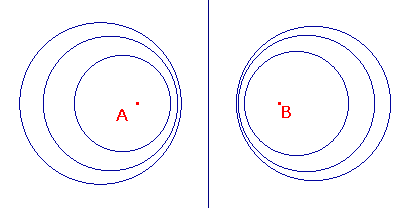Proof of Apollonius Theorem

 Apollonius Theorem Ak(A,B) is a line if k is 1, and a circle if k is not 1. If Ak(A,B) is the circle C with centre C and radius r, then A, B and C are collinear, with A and B on the same side of C, and CA.CB = r2. If A, B, and the circle C satisfy (1) and (2), then C, is in A(A,B).Proof We choose the perpendicular bisector of AB as the y-axis, and the line AB as the x-axis, so if A is (a,0), then B is (-a,0). Then P(x,y) lies on Ak(A,B) if and only if PA = k.PB. Squaring, we get (x-a)2 + y2 = k2{(x+a)2 + y2 }. Rearranging, we have (k2-1)(x2 + y2 + a2) + 2a(k2 +1)x = 0. If k = 1, this gives the line x = 0 (the perpendicular bisector of AB). Otherwise, we can divide by (k2 -1) to get the circle C : x2 + y2 + 2ax(k2+1)/(k2-1) + a2 = 0. For brevity, put K = (k2+1)/((k2-1). As k > 0, |K| > 1. Completing the square and simplifying, C has equation (x-aK)2+y2 = a2(K2-1), so has centre C (aK,0) and radius r where r2 = a2(K2-1). Thus, C lies on the x-axis, i.e. on AB, and, as |K| > 1, lies outside [A,B]. Also CA.CB = (a-aK)(-a-aK)=a2(K2-1) = r2. This proves the second part. Finally, suppose that A, B and the circle C satisfy (1) and (2). As A, B and C are collinear, C lies on the x-axis, so is (aK,0), for some K. As A and B lie on the same side of C, |K| > 1. Thus C has equation (x-aK)2 + y2 = r2. Also, by (2), r2 = CA.CB , so r2 =(a-aK).(-a-aK) = a2(K2-1). Combining these, C has equation x2 + y2 -2aKx + a2 = 0. Using the equation for Ak(A,B) found in the first part, C is in A(A,B) if and only if K = (k2+1)/(k2-1), i.e. k2 = (K+1)/(K-1). The left side is positive as |K| > 1, so k exists.